• Slides: 29GRAPHING A QUADRATIC FUNCTION A quadratic function has the form y = ax 2 + bx + c where a 0.GRAPHING A QUADRATIC FUNCTION The graph is “U-shaped” and is called a parabola.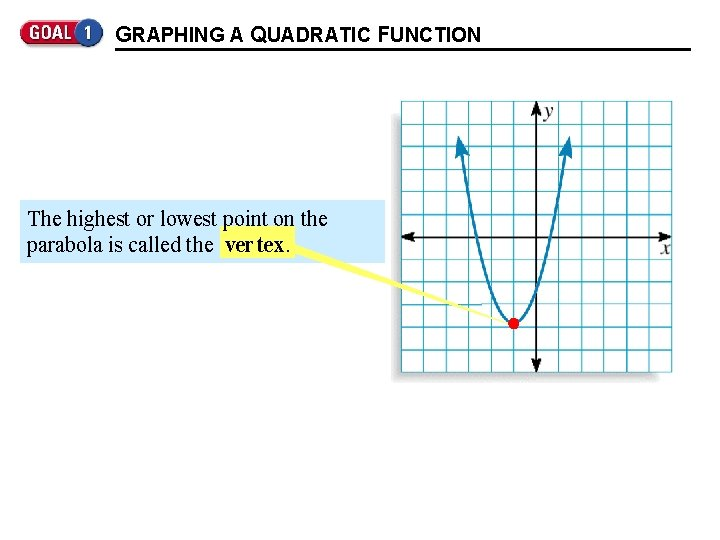GRAPHING A QUADRATIC FUNCTION The highest or lowest point on the parabola is called the ver tex.GRAPHING A QUADRATIC FUNCTION In general, the axis of symmetry for the parabola is the vertical line through the vertex.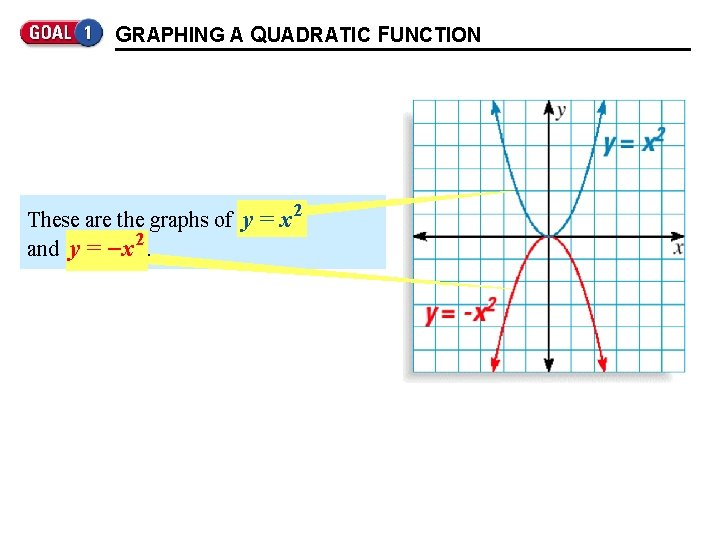GRAPHING A QUADRATIC FUNCTION These are the graphs of y = x 2 and y = - x. 2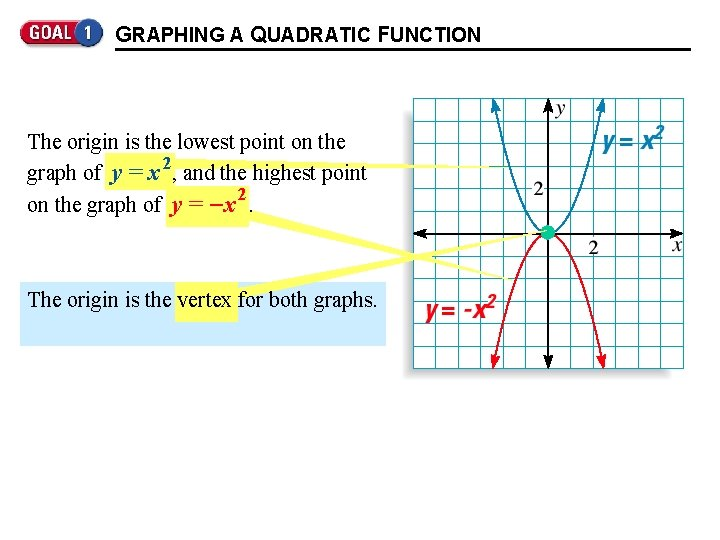GRAPHING A QUADRATIC FUNCTION The origin is the lowest point on the 2 graph of y = x , and the highest point 2 on the graph of y = - x. The origin is the vertex for both graphs.GRAPHING A QUADRATIC FUNCTION The y-axis is the axis of symmetry for both graphs.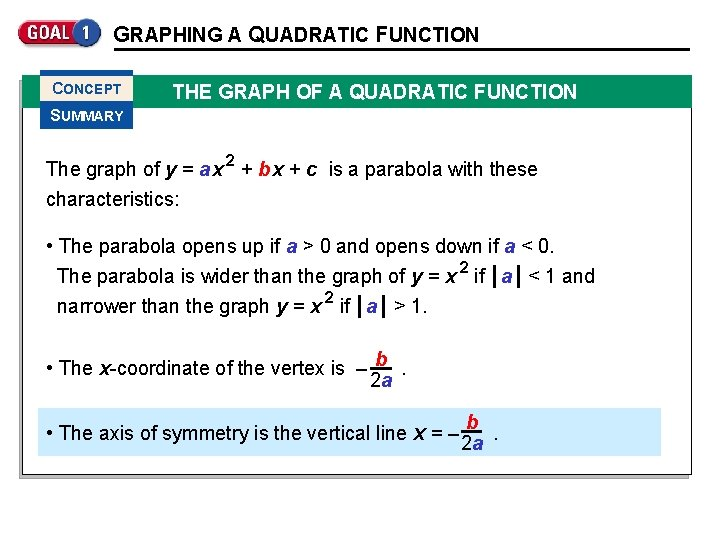GRAPHING A QUADRATIC FUNCTION CONCEPT THE GRAPH OF A QUADRATIC FUNCTION SUMMARY The graph of y = a x 2 + b x + c is a parabola with these characteristics: • The parabola opens up if a > 0 and opens down if a < 0. The parabola is wider than the graph of y = x 2 if a < 1 and narrower than the graph y = x 2 if a > 1. • The x-coordinate of the vertex is – b. 2 a b • The axis of symmetry is the vertical line x = – 2 a.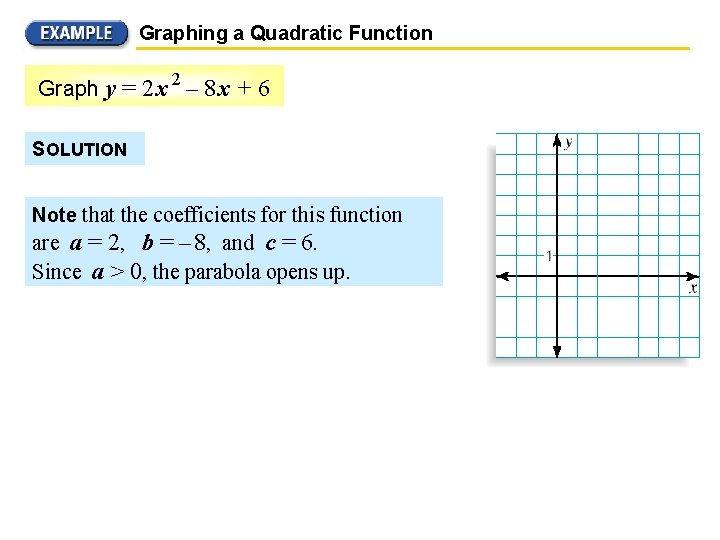Graphing a Quadratic Function 2 Graph y = 2 x – 8 x + 6 SOLUTION Note that the coefficients for this function are a = 2, b = – 8, and c = 6. Since a > 0, the parabola opens up.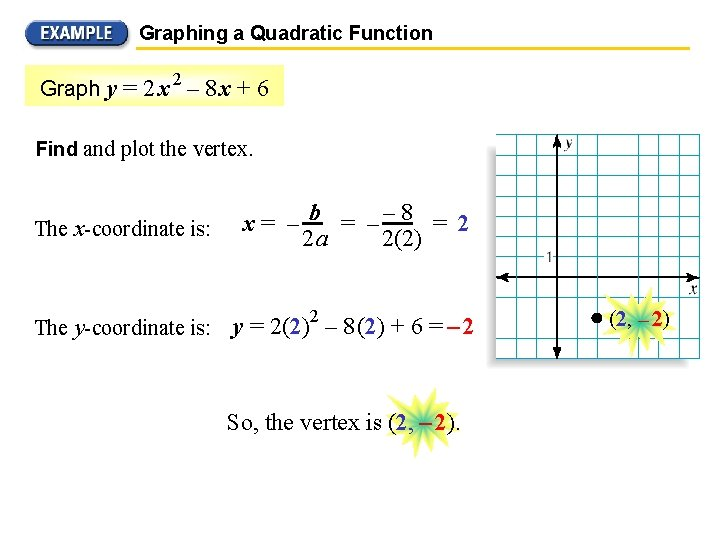Graphing a Quadratic Function 2 Graph y = 2 x – 8 x + 6 Find and plot the vertex. The x-coordinate is: x = – b = –– 8 = 2 2(2) 2 a The y-coordinate is: y = 2(2)2 – 8 (2) + 6 = – 2 So, the vertex is (2, – 2)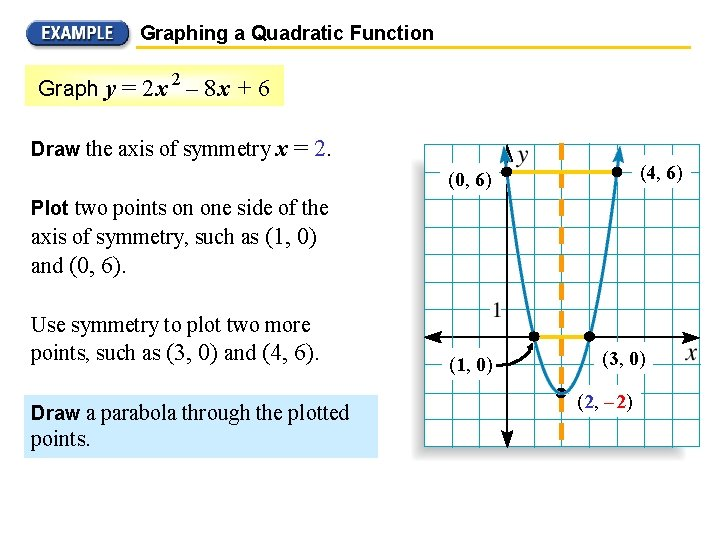Graphing a Quadratic Function 2 Graph y = 2 x – 8 x + 6 Draw the axis of symmetry x = 2. (4, 6) (0, 6) Plot two points on one side of the axis of symmetry, such as (1, 0) and (0, 6). Use symmetry to plot two more points, such as (3, 0) and (4, 6). Draw a parabola through the plotted points. (1, 0) (3, 0) (2, – 2)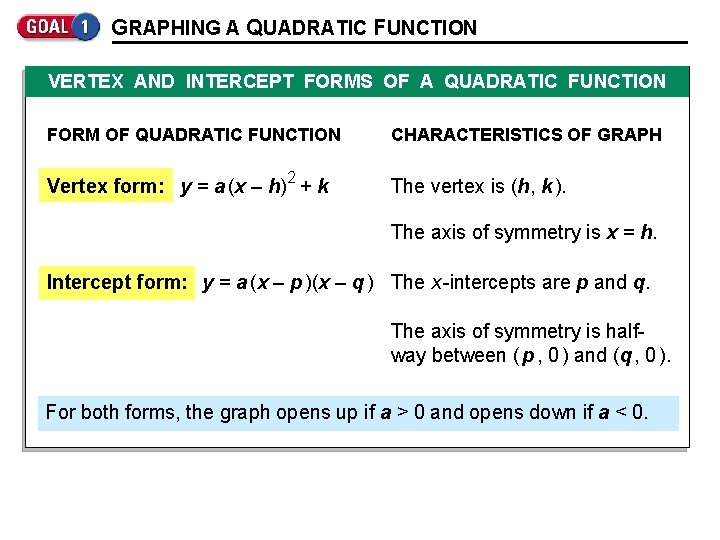GRAPHING A QUADRATIC FUNCTION VERTEX AND INTERCEPT FORMS OF A QUADRATIC FUNCTION FORM OF QUADRATIC FUNCTION CHARACTERISTICS OF GRAPH Vertex form: y = a (x – h)2 + k The vertex is (h, k ). The axis of symmetry is x = h. Intercept form: y = a (x – p )(x – q ) The x -intercepts are p and q. The axis of symmetry is halfway between ( p , 0 ) and (q , 0 ). For both forms, the graph opens up if a > 0 and opens down if a < 0.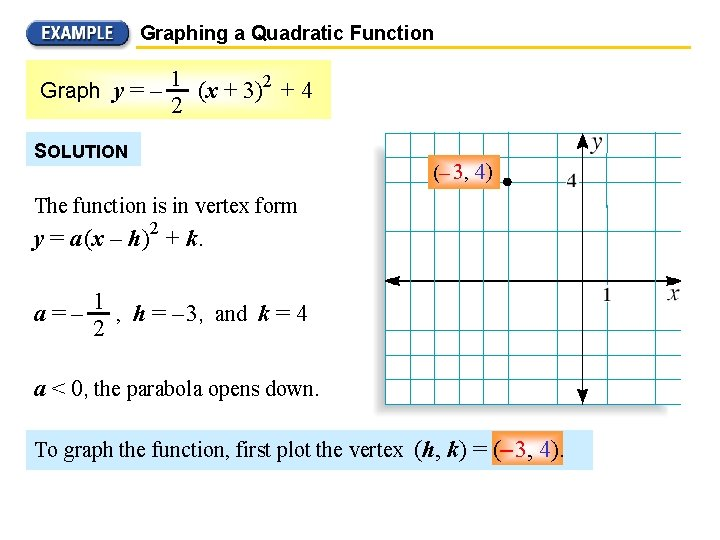Graphing a Quadratic Function Graph y = – 1 2 (x + 3)2 + 4 SOLUTION (– 3, 4) The function is in vertex form y = a (x – h)2 + k. a = – 1 , h = – 3, and k = 4 2 a < 0, the parabola opens down. To graph the function, first plot the vertex (h, k) = (– 3, 4).Graphing a Quadratic Function in Vertex Form Graph y = – 1 2 (x + 3)2 + 4 Draw the axis of symmetry x = – 3. (– 3, 4) (– 5, 2) (– 1, 2) Plot two points on one side of it, such as (– 1, 2) and (1, – 4). Use symmetry to complete the graph. (– 7, – 4) (1, – 4)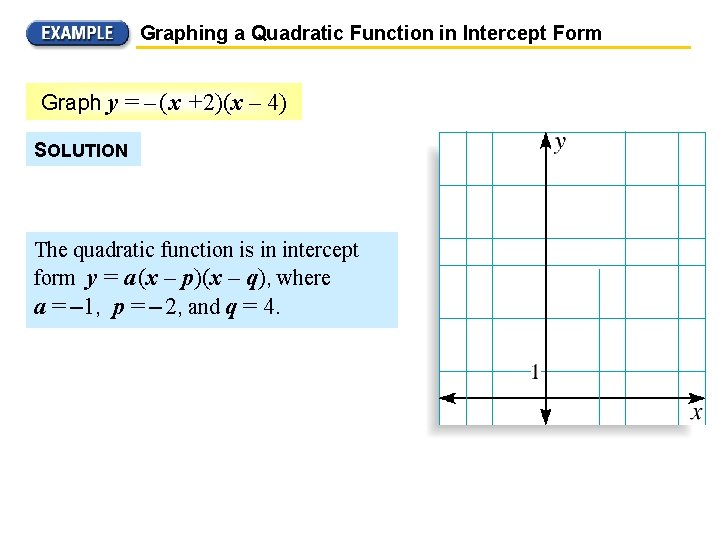Graphing a Quadratic Function in Intercept Form Graph y = – ( x +2)(x – 4) SOLUTION The quadratic function is in intercept form y = a (x – p)(x – q), where a = – 1, p = – 2, and q = 4.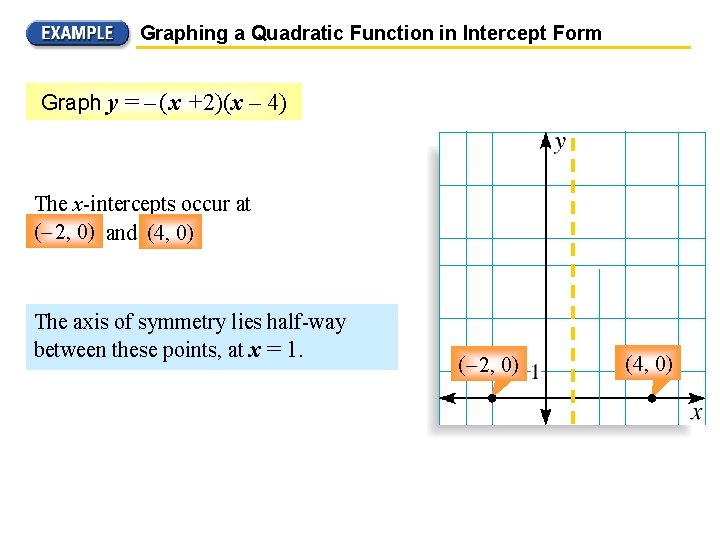Graphing a Quadratic Function in Intercept Form Graph y = – ( x +2)(x – 4) The x-intercepts occur at (– 2, 2, 0) 0) and (4, 0). The axis of symmetry lies half-way between these points, at x = 1. (– 2, 0) (4, 0)Graphing a Quadratic Function in Intercept Form Graph y = – ( x +2)(x – 4) (1, 9) So, the x-coordinate of the vertex is x = 1 and the y-coordinate of the vertex is: y = – (1 + 2) (1 – 4) = 9 (– 2, 0) (4, 0)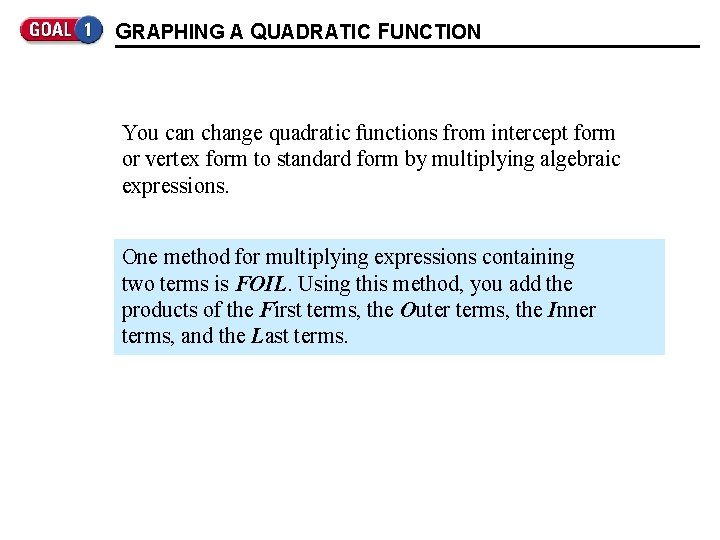GRAPHING A QUADRATIC FUNCTION You can change quadratic functions from intercept form or vertex form to standard form by multiplying algebraic expressions. One method for multiplying expressions containing two terms is FOIL. Using this method, you add the products of the First terms, the O uter terms, the Inner terms, and the Last terms.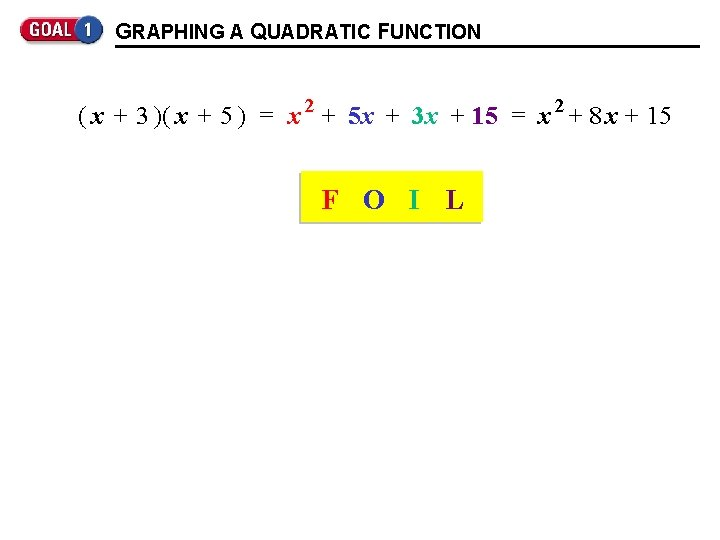GRAPHING A QUADRATIC FUNCTION ( x + 3 )( x + 5 ) = x 2 + 5 x + 3 x + 15 = x 2 + 8 x + 15 F O I L Inner First Last Outer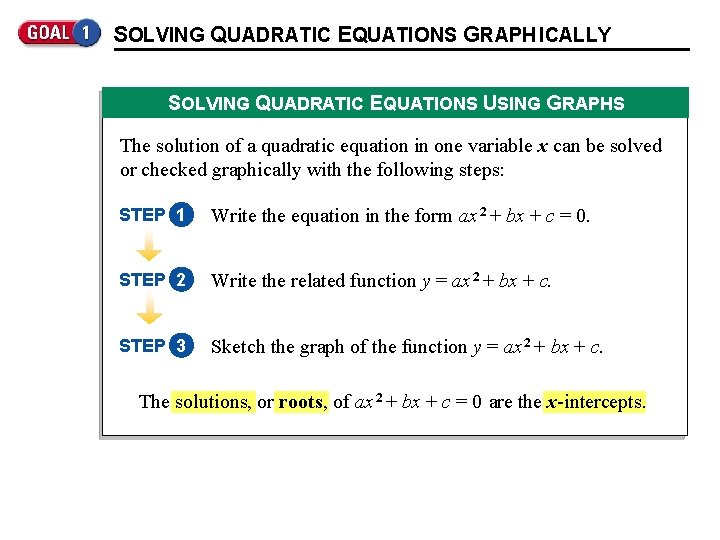SOLVING QUADRATIC EQUATIONS GRAPH ICALLY SOLVING QUADRATIC EQUATIONS USING GRAPHS The solution of a quadratic equation in one variable x can be solved or checked graphically with the following steps: STEP 1 Write the equation in the form ax 2 + bx + c = 0. STEP 2 Write the related function y = ax 2 + bx + c. STEP 3 Sketch the graph of the function y = ax 2 + bx + c. The solutions, or roots, of ax 2 + bx + c = 0 are the x-intercepts.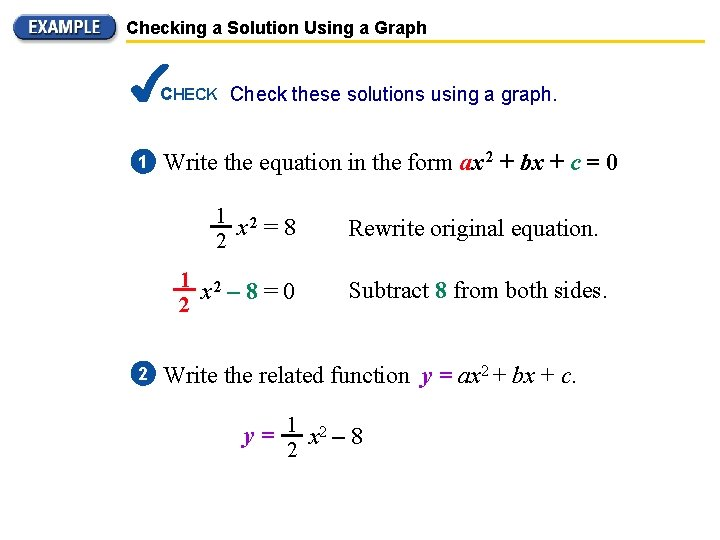Checking a Solution Using a Graph CHECK Check these solutions using a graph. 1 Write the equation in the form ax 2 + bx + c = 0 1 2 x =8 2 1 2 x – 8=0 2 2 Rewrite original equation. Subtract 8 from both sides. Write the related function y = ax 2 + bx + c. y = 1 x 2 – 8 2Checking a Solution Using a Graph Solve 1 2 x = 8 algebraically. 2 Check your solution graphically. SOLUTION 1 2 x = 8 2 CHECK Write original equation. x 2 = 16 Multiply each side by 2. x= 4 Find the square root of each side. Check these solutions using a graph.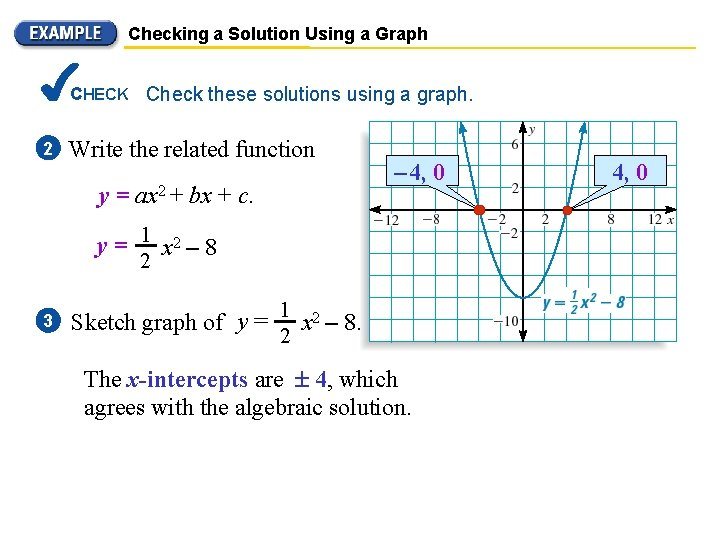Checking a Solution Using a Graph CHECK Check these solutions using a graph. 2 Write the related function y = ax 2 + bx + c. – 4, 0 y = 1 x 2 – 8 2 3 1 Sketch graph of y = x 2 – 8. 2 The x-intercepts are 4, which agrees with the algebraic solution. 4, 0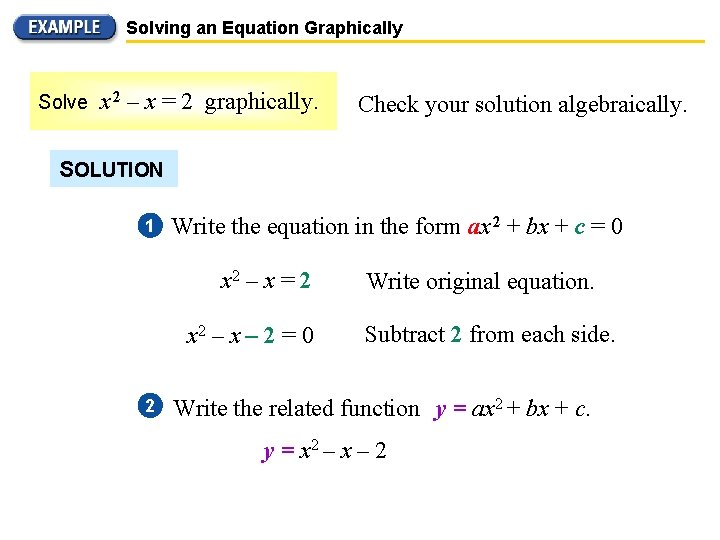Solving an Equation Graphically Solve x 2 – x = 2 graphically. Check your solution algebraically. SOLUTION 1 Write the equation in the form ax 2 + bx + c = 0 x 2 – x = 2 x 2 – x – 2 = 0 2 Write original equation. Subtract 2 from each side. Write the related function y = ax 2 + bx + c. y = x 2 – x – 2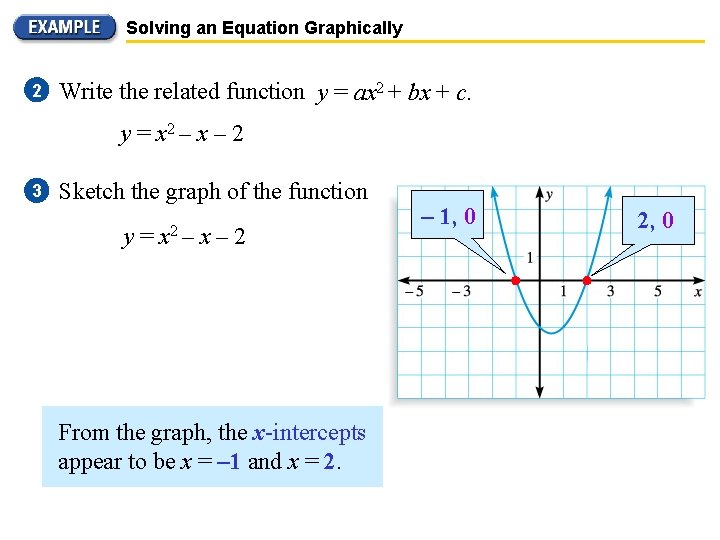Solving an Equation Graphically 2 Write the related function y = ax 2 + bx + c. y = x 2 – x – 2 3 Sketch the graph of the function y = x 2 – x – 2 From the graph, the x-intercepts appear to be x = – 1 and x = 2. – 1, 0 2, 0Solving an Equation Graphically From the graph, the x-intercepts appear to be x = – 1 and x = 2. – 1, 0 CHECK You can check this by substitution. Check x = – 1: Check x = 2: x 2 – x = 2 ? ? (– 1) = 2 22 – 2 = 2 1+1=2 4– 2=2 (– 1) 2 – 2, 0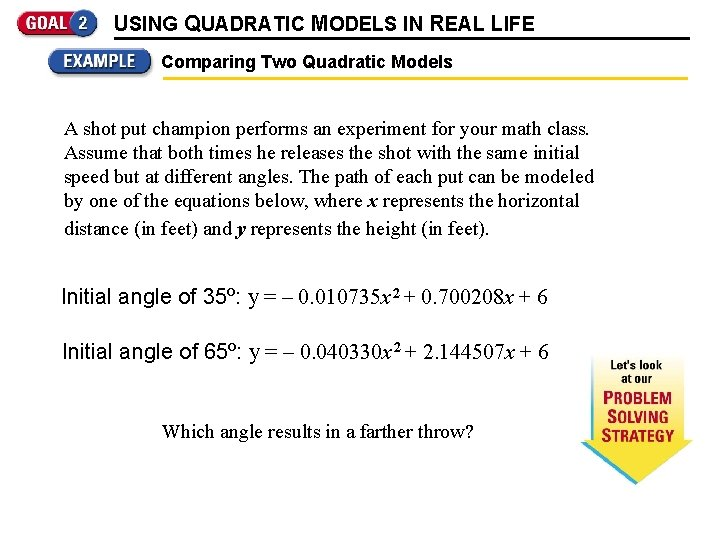USING QUADRATIC MODELS IN REAL LIFE Comparing Two Quadratic Models A shot put champion performs an experiment for your math class. Assume that both times he releases the shot with the same initial speed but at different angles. The path of each put can be modeled by one of the equations below, where x represents the horizontal distance (in feet) and y represents the height (in feet). Initial angle of 35º: y = – 0. 010735 x 2 + 0. 700208 x + 6 Initial angle of 65º: y = – 0. 040330 x 2 + 2. 144507 x + 6 Which angle results in a farther throw?Comparing Two Quadratic Models SOLUTION Begin by graphing both models in the same coordinate plane. Use only positive x-values because x represents the distance of the throw. Which angle results in a farther throw?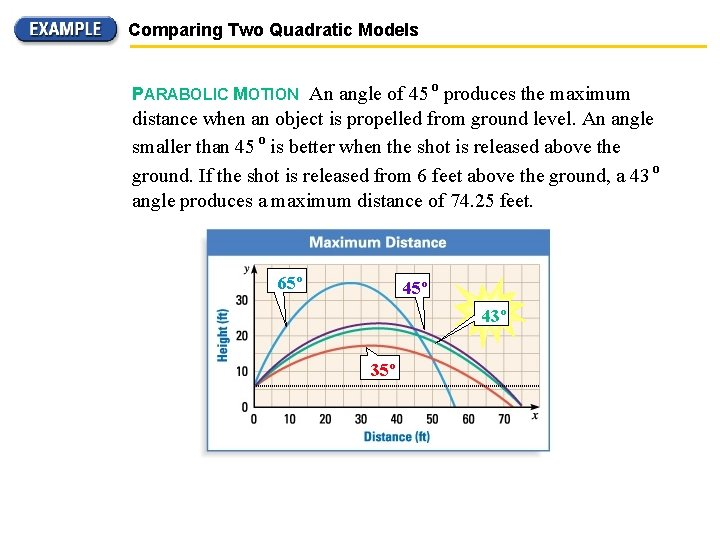Comparing Two Quadratic Models PARABOLIC MOTION An angle of 45 º produces the maximum distance when an object is propelled from ground level. An angle smaller than 45 º is better when the shot is released above the ground. If the shot is released from 6 feet above the ground, a 43 º angle produces a maximum distance of 74. 25 feet. 65º 43º 35º